# Market closed - page 2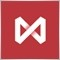10736

I think we should change the trading time check like this:

bool CheckExchTime()
{
MqlTick cur_tick;
MqlDateTime cur_time;
if(CopyTicks(Symbol(), cur_tick, COPY_TICKS_INFO, 0, 1)==1)
{
TimeToStruct(cur_tick.time, cur_time);
ulong trade_time = cur_time.hour * 3600 + cur_time.min * 60 + cur_time.sec;
{
return(true);
}
}
return(false);
}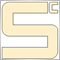8991

Sergei!

Read the TimeCurrent help - it says that this is the SERVER time

Возвращает последнее известное время сервера, время прихода последней котировки по одному из выбранных в "Обзоре рынка" символов.

If there are no ticks, weekends or non-trading time, TimeCurrent does not change.

It's easy to check this by running the Expert Advisor:

//+------------------------------------------------------------------+
int OnInit()
{
//--- create timer
EventSetTimer(1);
return(INIT_SUCCEEDED);
}
//+------------------------------------------------------------------+
void OnDeinit(const int reason)
{
//--- destroy timer
EventKillTimer();
}
//+------------------------------------------------------------------+
void OnTimer()
{
Comment(
"\n TimeCurrent: ",TimeCurrent(),
"\n TimeLocal: ",TimeLocal(),
"");
}
//+------------------------------------------------------------------+

When computer time changesTimeTradeServer also changes and shows computer timeTimeLocal.10736

Sergey Chalyshev:

If there are no ticks, weekends or non-trading times, TimeCurrent does not change.

AndTimeTradeServerdoes not work, it shows local computer time.

It is easy to check this by running the EA:

//+------------------------------------------------------------------+
int OnInit()
{
//--- create timer
EventSetTimer(1);
return(INIT_SUCCEEDED);
}
//+------------------------------------------------------------------+
void OnDeinit(const int reason)
{
//--- destroy timer
EventKillTimer();
}
//+------------------------------------------------------------------+
void OnTimer()
{
Comment(
"\n TimeCurrent: ",TimeCurrent(),
"\n TimeLocal: ",TimeLocal(),
"");
}
//+------------------------------------------------------------------+

When computer time changesTimeTradeServer also changes and shows computer timeTimeLocal.

What prevents you from checking the computer time via TimeTradeServer first, and then (if the time is correct) use

function I wrote above to more accurately check the time?

Although, of course, this is not the solution, because there may be no quotes for this symbol :(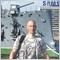7143

//+------------------------------------------------------------------+
//|                                                       test03.mq5 |
//|                                                   Sergey Gritsay |
//|                         https://www.mql5.com/ru/users/sergey1294 |
//+------------------------------------------------------------------+
#property version   "1.00"
//+------------------------------------------------------------------+
//| Script program start function                                    |
//+------------------------------------------------------------------+
void OnStart()
{
//---
if(CheckExchTime())Print("Торговля разрешена");
else Print("Торговля запрещена");
}
//+------------------------------------------------------------------+
bool CheckExchTime()
{
MqlTick last_tick;
MqlDateTime last_time;
MqlDateTime start_time;
MqlDateTime end_time;
datetime start=0;
datetime end=0;

ResetLastError();
if(SymbolInfoTick(_Symbol,last_tick))
{
TimeToStruct(last_tick.time,last_time);
Print(last_tick.time,": Bid = ",last_tick.bid," Ask = ",last_tick.ask," Last = ",last_tick.last,"  Volume = ",last_tick.volume);
{
start=__DATE__+(start_time.hour*60+start_time.min)*60;
end=__DATE__+(end_time.hour*60+end_time.min)*60;
Print(start," - ",end);
if(start==end)return(true);
if(last_tick.time>start && last_tick.time<end)return(true);
}
else Print("SymbolInfoSessionTrade(0) failed, error = ",GetLastError());
{
start=__DATE__+(start_time.hour*60+start_time.min)*60;
end=__DATE__+(end_time.hour*60+end_time.min)*60;
Print(start," - ",end);
if(start==end)return(true);
if(last_tick.time>start && last_tick.time<end)return(true);
}
else Print("SymbolInfoSessionTrade(1) failed, error = ",GetLastError());
}
else Print("SymbolInfoTick() failed, error = ",GetLastError());
return(false);
}
//+------------------------------------------------------------------+

...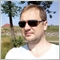13307

You guys are the developers!

When are you going to synchronise the terminal time with the Exchange time?

Mikalas, this is definitely not a question for the developers. That's what the broker admins do. You have to aim at them :-))7143

A little more tinkering with the code has resulted in this.

//+------------------------------------------------------------------+
//|                                                       test06.mq5 |
//|                                                   Sergey Gritsay |
//|                         https://www.mql5.com/ru/users/sergey1294 |
//+------------------------------------------------------------------+
#property version   "1.00"
//+------------------------------------------------------------------+
//| Expert initialization function                                   |
//+------------------------------------------------------------------+
int OnInit()
{
//--- create timer
EventSetMillisecondTimer(1);

//---
return(INIT_SUCCEEDED);
}
//+------------------------------------------------------------------+
//| Expert deinitialization function                                 |
//+------------------------------------------------------------------+
void OnDeinit(const int reason)
{
//--- destroy timer
EventKillTimer();

}
//+------------------------------------------------------------------+
//| Expert tick function                                             |
//+------------------------------------------------------------------+
void OnTick()
{
//---

}
//+------------------------------------------------------------------+
//| Timer function                                                   |
//+------------------------------------------------------------------+
void OnTimer()
{
//---
string text=NULL;
int symbol_total=SymbolsTotal(true);

for(int i=0; i<symbol_total; i++)
{
string symbol=SymbolName(i,true);
if(CheckExchTime(symbol,TimeCurrent()))text+="\n"+symbol+": Торговля разрешена";
else text+="\n"+symbol+": Торговля запрещена";
}
Comment(text);
}
//+------------------------------------------------------------------+
bool CheckExchTime(string symbol,datetime times)
{
MqlDateTime last_time;
MqlDateTime start_time;
MqlDateTime end_time;
datetime start=0;
datetime end=0;

ResetLastError();
datetime expiration=(datetime)SymbolInfoInteger(symbol,SYMBOL_EXPIRATION_TIME);
if(expiration!=0 && times>=expiration)return(false);
TimeToStruct(times,last_time);
{
start=StringToTime((string)last_time.year+"."+(string)last_time.mon+"."+(string)last_time.day+" "+(string)start_time.hour+":"+(string)start_time.min+":00");
end=StringToTime((string)last_time.year+"."+(string)last_time.mon+"."+(string)last_time.day+" "+(string)end_time.hour+":"+(string)end_time.min+":00");
if(start==end)return(true);
if(times>start && times<end)return(true);
}
//else Print("SymbolInfoSessionTrade(0) failed, error = ",GetLastError());
{
start=StringToTime((string)last_time.year+"."+(string)last_time.mon+"."+(string)last_time.day+" "+(string)start_time.hour+":"+(string)start_time.min+":00");
end=StringToTime((string)last_time.year+"."+(string)last_time.mon+"."+(string)last_time.day+" "+(string)end_time.hour+":"+(string)end_time.min+":00");
if(start==end)return(true);
if(times>start && times<end)return(true);
}
//else Print("SymbolInfoSessionTrade(1) failed, error = ",GetLastError());
return(false);
}
//+------------------------------------------------------------------+

Result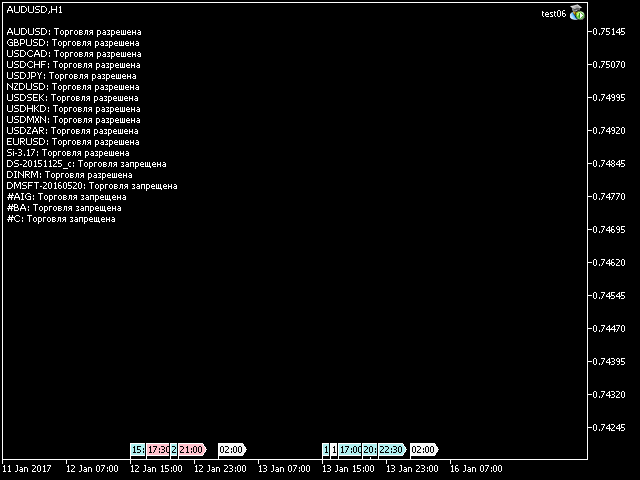10736

Sergey Gritsay:

A little more tinkering with the code has resulted in this.

Do you have any idea what you're talking about?7143

Do you have any idea what we are talking about?
Maybe I don't really understand it, I understand how you need a solution to avoid being caught at market closures outside exchange trading hours.10736

Sergey Gritsay:
Maybe I don't quite get it, I understand how, I need a solution to avoid market closures outside of exchange trading hours.

The solutions are not from the programmers, but from the developers (or broker, if they, the broker, are responsible for it)

The code you cited does not check the current time of the Exchange.10736

So far, settled on this solution

{
if ( ( tick_time.day_of_week == int(FirstDay)) ||
( tick_time.day_of_week == int(SecondDay)))//выходные
{
return(false);
}
#ifdef DEBUG
if ((tick_time.hour >= 0) && (tick_time.hour < 6))   // DEBUG 6-00
{
return(false);
}
#else

if ((tick_time.hour >= 0) && (tick_time.hour < 10))
{
return(false);
}
#endif
// 13 * 3600 + 59 * 60 + 30 = 50370 - 13:59:30
// 14 * 3600                = 50400 - 14:00:00
// 14 * 3600 + 30           = 50430 - 14:00:30
// 14 * 3600 + 60           = 50460 - 14:01:00

// 18 * 3600 + 44 * 60 + 30 = 67470 - 18:44:30
// 18 * 3600 + 45 * 60      = 67500 - 18:45:00
// 18 * 3600 + 45 * 60 + 30 = 67530 - 18:45:30
// 18 * 3600 + 46 * 60      = 67560 - 18:46:00

// 19 * 3600                = 68400 - 19:00:00
// 19 * 3600 + 60           = 68460 - 19:01:00

// 23 * 3600 + 49 * 60 + 30 = 85770 - 23:49:30
// 23 * 3600 + 50 * 60      = 85800 - 23:50:00
// 23 * 3600 + 50 * 60 + 30 = 85830 - 23:50:30
// 23 * 3600 + 51 * 60      = 85860 - 23:51:00
//---
lk_time = TimeCurrent(tick_time);
ulong trade_time = tick_time.hour * 3600 + tick_time.min * 60 + tick_time.sec;
//---                    //10:00:02
{
return(true);
}

return(false);

}

First I takeTimeTradeServer - I get the current "dirty" time

I check for a weekend and a time (trading) that "overlaps" all sessions,

and then usingTimeCurrent

Current server time, to check the time within sessions.

But this check has one major drawback.

If the local computer time is more than the server time, it will be fine, but

But if the local time is less, then we have a big problem.

If we miss the start of bidding for the difference between the trading server time and local time :(

That's why we needTimeCurrent

always return the server time, not only the time of the last known quote.